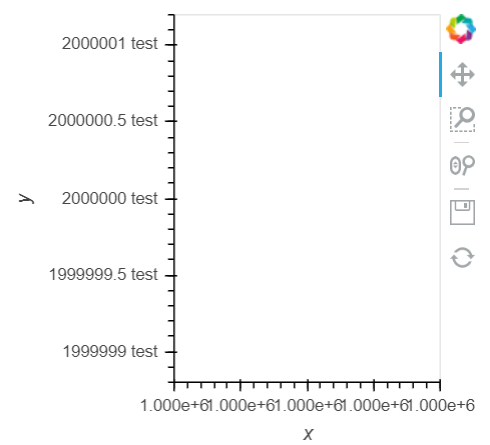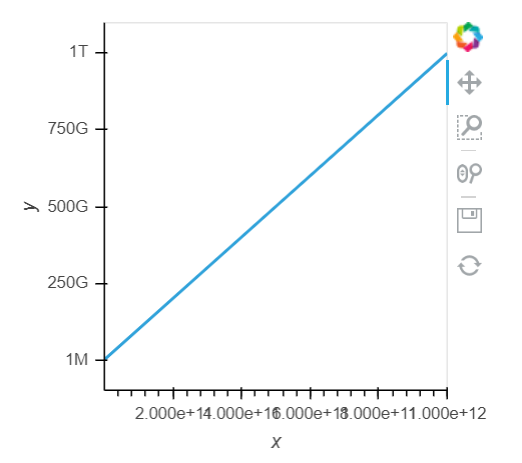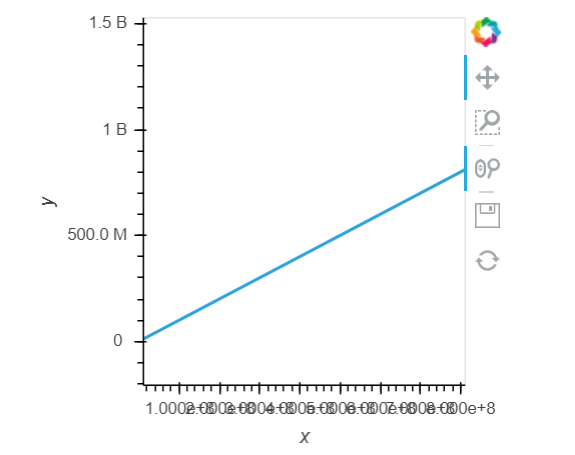# Axis formatter bug?

Hi,

I wanted to change the yaxis formatter for a holoviews plot, but no plots are returned if the formatter is slightly more complex than the example in the docs.

Code that works:

``````def formatter(value):
return str(value)+" test"

hv.Curve([[1e6,2e6,3e6],[1e6,2e6,3e6]]).opts(yformatter=formatter)
``````Code that doesn’t work:

``````from engineering_notation import EngNumber
def formatter(value):
return EngNumber(value)

hv.Curve([[1e6,2e6,3e6],[1e6,2e6,3e6]]).opts(yformatter=formatter)
``````

(blank output)

It also even breaks when you try to `return np.float64(value)`.

Any workaround?

I figured out a workaround with yticks:

``````from engineering_notation import EngNumber
a = hv.Curve(data = pd.DataFrame({'x':[1e6,1e9,1e12],'y':[1e6,1e9,1e12]}))
b = list(a.range('y'))
n_ticks = 5
a.opts(yticks=[(i,EngNumber(i)) for i in np.linspace(*(b+[n_ticks]))])
``````But this is not an ideal solution, as the ticks don’t change if you zoom in…

Ah!

You need to use js with FuncTickFormatter:

``````from bokeh.models import FuncTickFormatter
formatter = FuncTickFormatter(code='''
var space = ' ';

// Giga
if(tick >= 1e10)
return (tick / 1e9).toFixed(1) + space + 'B';
else if(tick >= 1e9)
return (tick / 1e8).toFixed(0) / 10 + space + 'B';

// Mega
else if(tick >= 1e7)
return (tick / 1e6).toFixed(1) + space + 'M';
else if(tick >= 1e6)
return (tick / 1e5).toFixed(0) / 10 + space + 'M';

// Kilo
else if(tick >= 1e4)
return (tick / 1e3).toFixed(1) + space + 'k';
else if(tick >= 1e3)
return (tick / 1e2).toFixed(0) / 10 + space + 'k';

// Unit
else if(tick >= 1e1)
return (tick / 1e0).toFixed(0) + space + '';
else
return (tick / 1e-1).toFixed(0) / 10 + space + '';
''')

hv.Curve([[1e6,2e6,3e6],[1e6,2e6,3e6]]).opts(yformatter=formatter)
``````

I don’t know js, so I just copied from here and swapped ‘number’ for ‘tick’:

Looks like this: